Function Repository Resource:

# MultiplicativePersistence

Compute the multiplicative persistence, in any base, of an integer

Contributed by: Christopher Stover
 ResourceFunction["MultiplicativePersistence"][n,b] gives the multiplicative persistence of the non-negative integer n when expressed in the base b. ResourceFunction["MultiplicativePersistence"][n] gives the base-10 muliplicative persistence of n.

## Details

Starting with a non-negative integer n, multiply its base-b digits, then multiply the digits of the resulting number, etc., until the result has only one digit. The number of multiplications required to reach the single digit stopping point is called the multiplicative persistence of n.
As an example in base 10, consider n=14691:
Multiplying its digits yields 1×4×6×9×1=216.
Multiplying the digits of the result yields 2×1×6=12.
Multiplying the digits of the result yields 1×2=2. Because 2 is a single-digit number, the process stops.
Because it took three steps to reach the stopping point, ResourceFunction["MultiplicativePersistence"] returns 3.
The multiplicative persistence of a number is also sometimes called its number length.

## Examples

### Basic Examples (2)

Compute the multiplicative persistence of 14691:

 In:=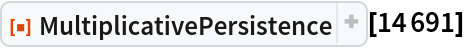Out=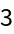Compute the multiplicative persistence of 14691, base-14:

 In:=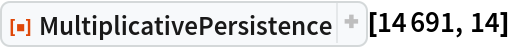Out=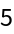### Scope (2)

 In:=Out=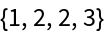In:=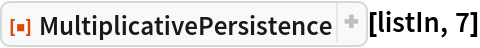Out=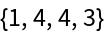Compute the multiplicative persistence of the first 100 integers (OEIS A031346):

 In:=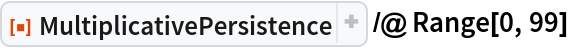Out=### Properties and Relations (3)

The result returned by MultiplicativePersistence can be iteratively computed using NestWhileList:

 In:=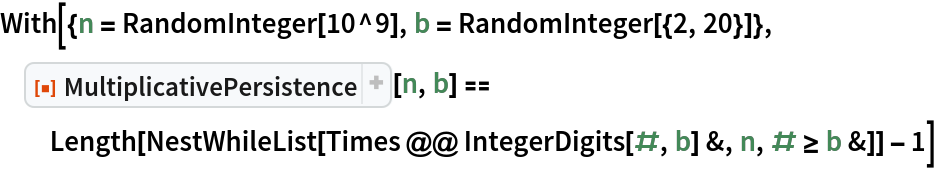Out=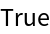The single-digit integer that marks the end of the digit multiplication process is called the multiplicative digital root and is returned by the resource function MultiplicativeDigitalRoot:

 In:=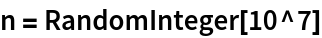Out=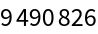In:=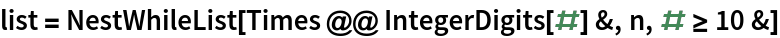Out=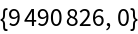In:=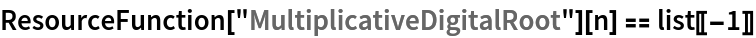Out=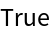The additive analogue of multiplicative persistence is called additive persistence and is returned by the resource function AdditivePersistence:

 In:=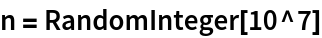Out=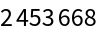In:=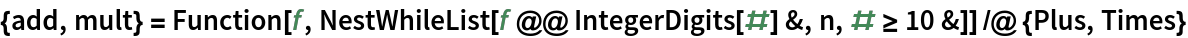Out=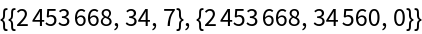In:=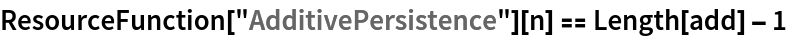Out=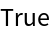### Possible Issues (1)

MultiplicativePersistence requires its input to be non-negative:

 In:=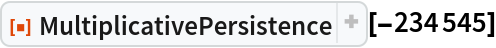Out=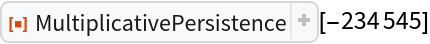### Neat Examples (1)

The smallest integers with multiplicative persistences equal to 0,1,2,3,4, (OEIS A003001) are:

 In:=Out=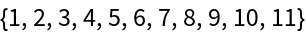It is known that there is no number N<10233 with multiplicative persistence larger than 11 (Carmody 2001).

## Version History

• 1.1.0 – 25 May 2023
• 1.0.0 – 09 August 2022